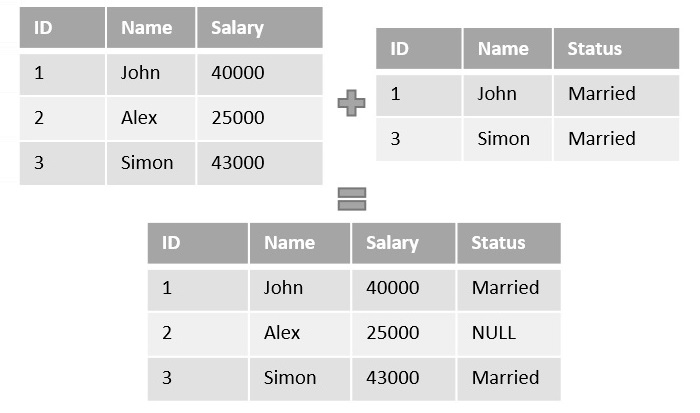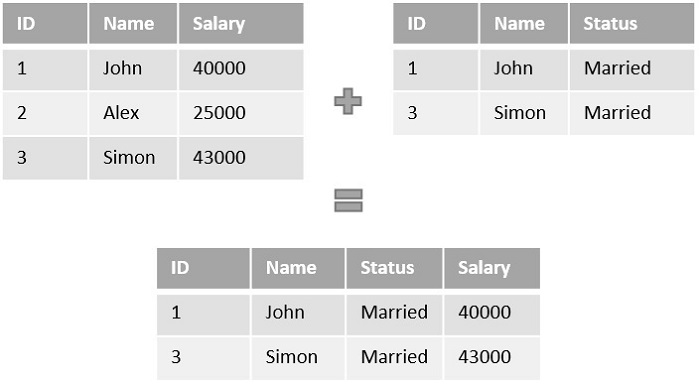# Left Join vs Right Join

The main difference between the Left Join and Right Join can be observed in the way tables are joined.

They are both types of Outer Joins; that is, they retain unmatched rows in one table and discard the unmatched rows of another. Left Join preserves the unmatched rows of left table while Right join preserves the unmatched rows of right table.

## Working of Left Join

Left Join or Left Outer Join in SQL combines two or more tables, where the first table is returned wholly; but, only the record(s) that have counterparts in first table are returned from consequent tables.

If the ON clause matches 0 (zero) records in consequent tables with the rows in first table, left join will still return these rows of first table in the result, but with NULL in each column from the right table.

### Syntax

Following is the basic syntax of Left Join in SQL −

```SELECT table1.column1, table2.column2...
FROM table1
LEFT JOIN table2
ON table1.column_name = table2.column_name;
```

### Example

The example below demonstrates the Left Join operation on two relative tables. Here, the first table contains the salary information while the second table contains marital status information. Since Alex’s status is unknown, it is not recorded in the table.When both tables are joined using the Left Join query, since there is no record matching Alex’s Status, the value is recorded as NULL in the final table.

## Working of Right Join

Right Join or Right Outer Join query in SQL returns all rows from the right table, even if there are no matches in the left table. This means that if the ON clause matches 0 (zero) records in left table with the records in right table; right join will still return the rows of right table in the result, but with a NULL value in each column of the left table.

### Syntax

Following is the basic syntax of a Right Join in SQL −

```SELECT table1.column1, table2.column2...
FROM table1
RIGHT JOIN table2
ON table1.column_name = table2.column_name;
```

### Example

Now in this example, let us try to perform the Right Join operation on the same tables. Here, we are starting the join from the right table; since, the right table does not contain the record value matching Alex’s row, the row is discarded from the final table.The final table only consists of two rows as the right table consists of two rows only.

## Left Join Vs Right Join

Let us summarize all the differences between the Left Join and Right Join in the table below −

Left Join Right Join
Left Join matches the data of the first table or the left table with the data in second table. If the data is matched, the records are combined; otherwise, NULL is recorded.Right Join matches the data of the second table or right table with the data in first table. If the data is matched, the records are combined; otherwise, NULL is recorded.
If the first table has less rows than the second table, extra unmatched rows from the second table are discarded. If the second table has less rows than the first table, extra unmatched rows from the first table are discarded.
This Join is also known as Left Outer Join This Join is also known as Right Outer Join
*= is used in Transact SQL, instead of using the LEFT JOIN or LEFT OUTER JOIN query. =* is used in Transact SQL, instead of using the RIGHT JOIN or RIGHT OUTER JOIN query.

As we can observe from the summary, there aren’t wide range of differences between the Left and Right joins. Every difference between them zeroes down to the way the tables are joined and the join point of view.